Courses

# NCERT Solutions(Part- 1)- Linear Equations in One Variable Class 8 Notes | EduRev

## Class 8 Mathematics by VP Classes

Created by: Vp Classes

## Class 8 : NCERT Solutions(Part- 1)- Linear Equations in One Variable Class 8 Notes | EduRev

The document NCERT Solutions(Part- 1)- Linear Equations in One Variable Class 8 Notes | EduRev is a part of the Class 8 Course Class 8 Mathematics by VP Classes.
All you need of Class 8 at this link: Class 8

Ques: Solve the following equations.
1. x – 2 = 17
2. y + 3 = 10
3. 6 = z + 2
4.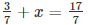5. 6x = 12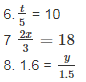9. 7x – 9 = 16
10. 14y – 8 = 13
11. 17 + 6p = 9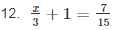Ans:
1. x – 2 = 7
Transposing (–2) to RHS, we have
x = 7 + 2
∴ x = 9

2. y + 3 = 10
Transposing 3 to RHS, we have
y = 10 – 3
∴ y = 7

3. 6 = z + 2
Transposing 2 to LHS, we have

6 – 2 = z
4 = z
∴ z = 4

4.Transposing 3/7 to RHS, we have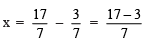= 14/7
= 2

5. 6x = 12
Dividing both sides by 6, we have: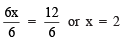∴ x = 2

6. t/5 = 10
Multiplying both sides by 5, we have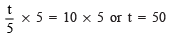∴ t = 50

7. 2x/3 = 18

Multiplying both sides by 3, we have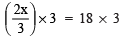⇒ 2x = 54

Dividing both sides by 2, we have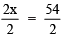x = 54/2 = 27

∴ x = 27
8. 1.6 =  y/1.5

Multiplying both sides by 1.5, we have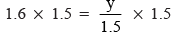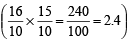2.4 = y
∴ y = 2.4

9. 7x – 9 = 16
Transposing (–9) to RHS, we have
7x = 16 + 9 = 25

Dividing both sides by 7, we have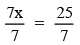∴ x = 25/7

10. 14y – 8 = 13
Transposing –8 to RHS, we have
14y = 13 + 8 = 21
Dividing both sides by 14, we have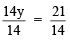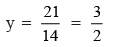∴ y = 3/2

11. 17 + 6p = 9
Transposing 17 to RHS, we have
6p = 9 – 17 = –8
Dividing both sides by 6, we have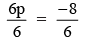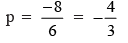∴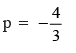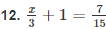Transposing 1 to RHS, we have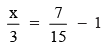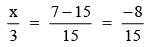Multiplying both sides by 3, we have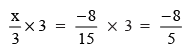∴ x = -8/5

93 docs|16 tests

,

,

,

,

,

,

,

,

,

,

,

,

,

,

,

,

,

,

,

,

,

;